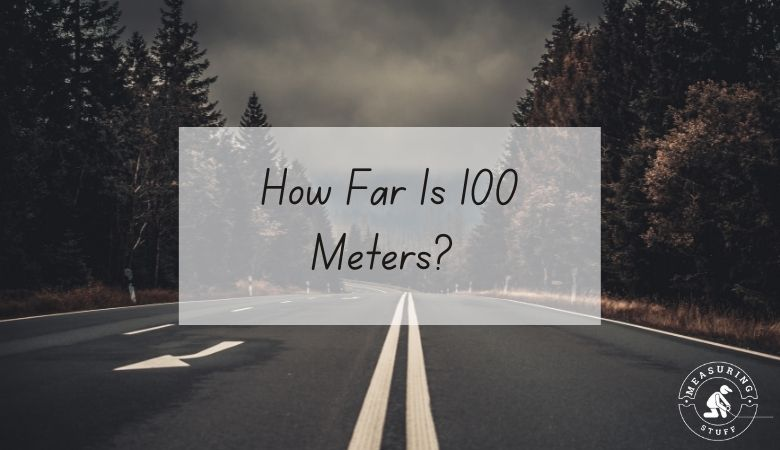# How Far Is 100 Meters? (With Visuals)

Last updated on June 27th, 2022 at 06:29 pm

If you are looking to find out how far 100 meters is, this article will show you along with some visual examples as a reference.

100 meters is not a far distance and is easy to see visually.

Measuring this distance with a standard measuring tool such as a measuring tape is not very common although it can be done.

To get a sense of how far 100 meters is, you can reference items of similar length or distance to give you an idea.

Here are some examples.

100 meters is equal to 328 feet or 109.36 yards.

100 meters is also equal to 1/10 of a kilometer which is 1000 meters.

## NFL Football field

One of the most common items to reference when determining distance is a football field.

If you are a football fan you most likely know how big a football field is visually.

An NFL football field is 360 feet long which is equal to 109.73 meters including the end zones.

Although it’s not exactly 100 meters long, a football field is a great example to use as a reference for how far 100 meters is.

You can also reference the width of the field which is 160 feet or 48.76 meters.

Therefore picturing 2 fields placed side by side will give you another example of how far 100 meters is.

In professional basketball leagues like the NBA, the courts used have dimensions of 94 feet long x 50 feet wide.

This is equal to 28.65 meters long x 15.24 meters wide.

1 basketball court = 28.65 meters long.

3.5 basketball courts = 100.27 meters long.

Therefore if you visualize 3.5 basketball courts in length, this will give you a good representation of how long 100 meters really is.

## 5.5 Bowling lanes

Keeping with the sports theme, many people are familiar with the length of a bowling lane visually.

If you were to measure the length of a bowling lane from the foul line to the center pin, it would equal 60 feet long.

60 feet is equal to 18.28 meters.

CHECK OUT  What Are The Dimensions Of A Boxing Ring?

1 bowling lane = 18.28 meters long.

5.5 bowling lanes = 100.54 meters long.

Therefore if you visualize 5.5 bowling lanes in length, this will show you how far 100 meters is.

It also tells you that 5.5 bowling lanes equal the same length of 3.5 basketball courts.

## ¼ Empire state building height

The Empire State Building is one of the most famous structures in the world.

If you have ever visited this building in New York, you will know how tall it is.

From the ground to the tip, the Empire State Building stands 443 meters tall.

So to get a visual reference of how far 100 meters is, picture ¼ of the Empire State Building’s height.

## Airport runway

The runways used at airports around the world can vary in size.

Some runways are longer than others and some are wider than others.

The width of a runway used by commercial aircraft at international airports is normally between 150 and 200 feet wide which is equal to between 45 and 61 meters.

Therefore, if you look at the width of an airport runway it will be around half of 100 meters. Or you can say the width of 2 airport runways is equal to 100 meters as they are about 50 meters wide each.

## How long does it take to walk 100 meters?

Not everyone walks at the same speed so the time it takes to walk 100 meters will vary from person to person.

100 meters is not a far distance so it won’t take very long to walk.

On average, a person walks around 3 miles per hour which is 0.05 miles per minute.

100 meters is equal to 0.062 miles.

Therefore, a person will walk 100 meters in 1.24 minutes at a speed of 3 miles per hour.

## How do you convert 100 meters into yards?

You can easily convert 100 meters into yards by using a simple formula or by using a conversion calculator.

1 meter equals 1.093 yards which is the conversion factor.

You can use 1.093 as the conversion factor to convert any number of meters into yards.

For example:

100 meters x 1.093 (conversion factor) = 109.3 yards.

You can also use this online calculator to convert units of measurements including meters into yards and vice versa.